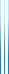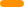# PublicationsPapers

## Spectral methods in extremal combinatorics

Yuval Filmus
PhD thesis, CMS Doctoral Prize

Extremal combinatorics studies how large a collection of objects can be if it satisfies a given set of restrictions. Inspired by a classical theorem due to Erdős, Ko and Rado, Sominovits and Sós posed the following problem: determine how large a collection of graphs on the vertex set $\{1,\ldots,n\}$ can be, if the intersection of any two of them contains a triangle. They conjectured that the largest possible collection, containing $1/8$ of all graphs, consists of all graphs containing a fixed triangle (a triangle-star). The first major contribution of the thesis is a confirmation of this conjecture. This result first appeared in our paper Triangle-intersecting families of graphs with David Ellis and Ehud Friedgut.

We prove the Simonovits–Sós conjecture in the following strong form: the only triangle-intersecting families of the maximal measure $1/8$ are triangle-stars (uniqueness), and every triangle-intersecting family of measure $1/8-\epsilon$ is $O(\epsilon)$-close to a triangle-star (stability). Our proof uses spectral methods (Hoffman’s bound).

In order to prove the stability part of our theorem, we utilize a structure theorem for Boolean functions on $\{0,1\}^m$ whose Fourier expansion is concentrated on the first $t+1$ levels, due to Kindler and Safra. The second major contribution of this thesis consists of two analogs of this theorem for Boolean functions on $S_m$ whose Fourier expansion is concentrated on the first two levels. These results appear in our papers A quasi-stability result for dictatorships in $S_n$ and A stability results for balanced dictatorships in $S_n$, both with David Ellis and Ehud Friedgut.

In the same way that the Kindler–Safra theorem is useful for studying triangle-intersecting families, our structure theorems are useful for studying intersecting families of permutations, which are families in which any two permutations agree on the image of at least one point. Using one of our theorems, we give a simple proof of the following result of Ellis, Friedgut and Pilpel: an intersecting family of permutations on $S_m$ of size $(1-\epsilon)(m-1)!$ is $O(\epsilon)$-close to a double coset, a family which consists of all permutations sending some point $i$ to some point $j$.

The thesis includes a detailed exposition of Friedgut’s paper On the measure of intersecting families, uniqueness and stabilitya proof of the Ahlswede–Khachatrian theorem in the $\mu_p$ setting, and a gentle introduction to the representation theory of $S_n$ from the point of view of class functions.

## BibTeX

@phdthesis{Filmus2013,
author = {Yuval Filmus},
title = {Spectral methods in extremal combinatorics},
school = {University of Toronto},
year = {2013}
}copy to clipboard